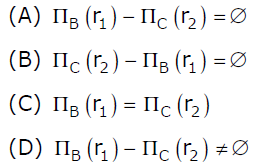GeeksforGeeks App
Open AppBrowser
Continue

# GATE | GATE CS 2012 | Question 41

Suppose (A, B) and (C,D) are two relation schemas. Let r1 and r2 be the corresponding relation instances. B is a foreign key that refers to C in r2. If data in r1 and r2 satisfy referential integrity constraints, which of the following is ALWAYS TRUE?

``

(A) A
(B) B
(C) C
(D) D